# Math in Focus Grade 2 Chapter 7 Practice 3 Answer Key Measuring in Centimeters

Practice the problems of Math in Focus Grade 2 Workbook Answer Key Chapter 7 Practice 3 Measuring in Centimeters to score better marks in the exam.

## Math in Focus Grade 2 Chapter 7 Practice 3 Answer Key Measuring in Centimeters

Check (✓) the correct way to measure the length of the pencil.

Question 1.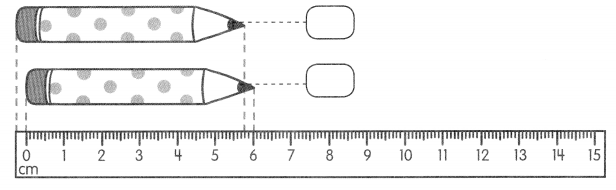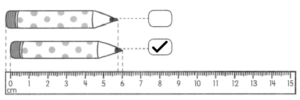Use your centimeter ruler to draw.

Question 2.
A line that is 5 centimeters long
___________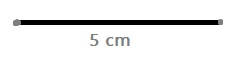Question 3.
A line that is 12 centimeters long
_____________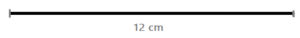Question 4.
A line that is 9 centimeters long
___________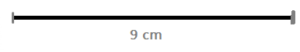Use your centimeter ruler to draw.

Question 5.
A line that is 6 centimeters long
_______________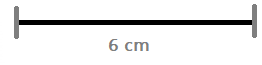Question 6.
A line that is 2 centimeters shorter than the line in Exercise 4.
_____________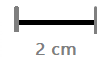Question 7.
A line that is 2 centimeters longer than the line in Exercise 5.
_____________Use a piece of string to find the length.

Question 8.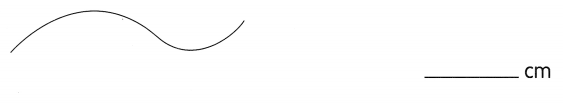Question 9.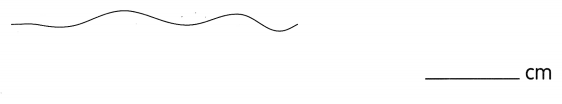Question 10.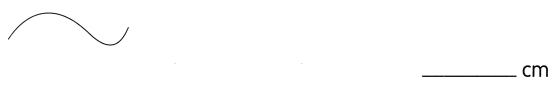Cut a piece of string as long as the drawing below. Then place the string on a centimeter ruler to find its length.

Question 11.
a. How long is the string? ___ cm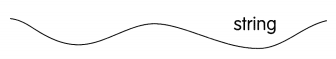Answer: The string is 8.5 cm long

b. This string is used to form the following shapes. Use a string and a centimeter ruler to measure each of them.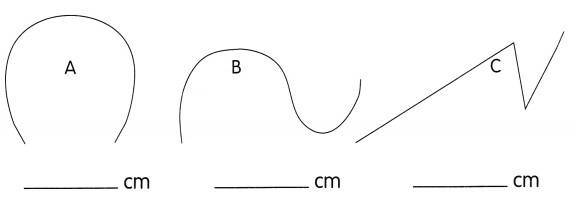Do they all have the same length? ____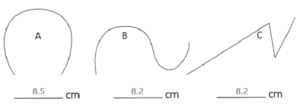Find the missing numbers.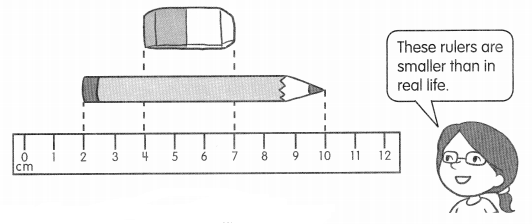Question 12.
The pencil is _________ centimeters long.
Answer: The pencil is 8 centimeters long.

Question 13.
The eraser is _________ centimeters long.
Answer: The eraser is 3 centimeters long.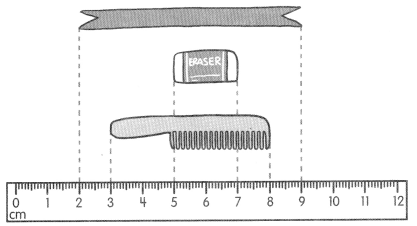Question 14.
The length of the comb is ___ centimeters.
Answer: The length of the comb is 5 centimeters

Question 15.
The length of the ribbon is ___ centimeters.
Answer: The length of the ribbon is 7 meters centimeters.

Question 16.
The length of the eraser is ____ centimeters.
Answer: The length of the eraser is 2 centimeters.